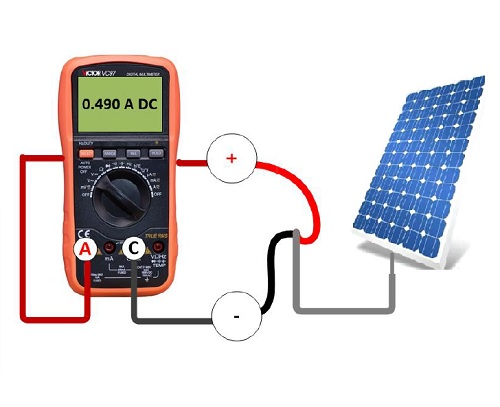## How to: Test Solar PanelOTHERS

#### #TAG Category

# Troubleshooting
# Wiring

#### Testing Solar Panel

1. Disconnect the Solar panel from the controller.

2. Connect a Voltmeter to the Solar panel two wires as described on image 1.

3. The measured voltage supposed to be close to 20 V DC.

4. Connect an Amper-meter to the Solar panel two wires as described on image 2.

5. The measured current can be calculated by the next formula: “I=P/V” where “I” is the current , “P” is the solar panel power, and “V” is the solar panel Voltage . For example, if the solar panel power is 10 Watts, the current supposed to be 10 watts/20 volts=0.5 A DC.

#### Testing Rechargeable battery charging

1. Connect a Voltmeter to the rechargeable battery two poles as described on image 3. The voltage supposed to be above 12 V DC.

2. Connect back the Charge limiter to the rechargeable battery.

3. Disconnect the Solar panel from the Charge limiter

4. Ensure that the battery voltage does not reduced. The voltage supposed to be stable.

5. Connect an Amper-meter to the rechargeable battery as described on image 4. In this way you can measure the charging current.